# Character recognition tutorial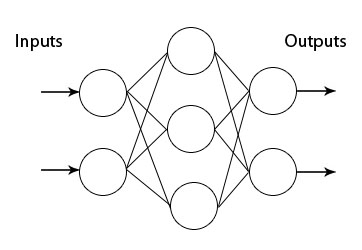Here we give an example using multi-layer perceptron for simple character recognition.

This is not a full-featured character recognition application.

Rather it is just an educational example to get an idea how to use the ANN toolbox in Scilab.

Our neural network will recognize two letters: T and U.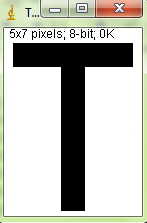```T = [...
1 1 1 1 1 ...
0 0 1 0 0 ...
0 0 1 0 0 ...
0 0 1 0 0 ...
0 0 1 0 0 ...
0 0 1 0 0 ...
0 0 1 0 0 ...
]';
```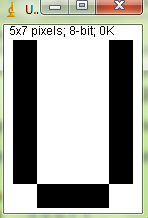```U = [...
1 0 0 0 1 ...
1 0 0 0 1 ...
1 0 0 0 1 ...
1 0 0 0 1 ...
1 0 0 0 1 ...
1 0 0 0 1 ...
0 1 1 1 0 ...
]';
```

These pictures are screenshots of *.pgm files opened with ImageJ.

The contents of the *.pgm files are

T.pgm

```P2
5 7
1
1 1 1 1 1
0 0 1 0 0
0 0 1 0 0
0 0 1 0 0
0 0 1 0 0
0 0 1 0 0
0 0 1 0 0
```

U.pgm

```P2
5 7
1
1 0 0 0 1
1 0 0 0 1
1 0 0 0 1
1 0 0 0 1
1 0 0 0 1
1 0 0 0 1
0 1 1 1 0
```

To recognize these letters, we built a perceptron with three layers.

The input layer has 35 neurons – one neuron for each pixel in the picture.

The output layer has two neurons – one neuron per class in our classification task (one for “T”, another one for “U”).

The hidden layer has 10 neurons.

The numbers of neurons in all layers are collected in a row vector

```N = [35 10 2];
```

Then, the weights of the connections between the neurons are initialized

```W = ann_FF_init(N);
```

Our training set consists of the two images above

```x = [T, U];
```

Now let’s specify the desired responses.

If the input layer reads “T” then the first output neuron should give 1 and the second output neuron should give 0

```t_t = [1 0]';
```

If the input layer reads “U” then the first output neuron should give 0 and the second output neuron should give 1

```t_u = [0 1]';
```

To be used in Scilab ANN routines, the responses need to be combined in a matrix that has the same amount of columns as the training set

```t = [t_t, t_u];
```

Other training parameters are the learning rate and the threshold of error

```lp = [0.01, 1e-4];
```

The number of training cycles:

```epochs = 3000;
```

The network is trained with the standard backpropagation in batch mode:

```W = ann_FF_Std_batch(x,t,N,W,lp,epochs);
```

`W` is the matrix of weights after the training.

Let’s test it:

```y = ann_FF_run(x,N,W)
disp(y)
```

It gave me:

```    0.9007601    0.1166854
0.0954540    0.8970468
```

Looks fine.

Now, let’s feed the network with slightly distorted inputs and see if it recognises them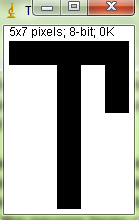```T_odd = [...
1 1 1 1 1 ...
0 0 1 0 1 ...
0 0 1 0 1 ...
0 0 1 0 0 ...
0 0 1 0 0 ...
0 0 1 0 0 ...
0 0 1 0 0 ...
]';
```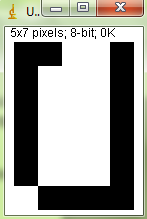```U_odd = [...
1 1 0 0 1 ...
1 0 0 0 1 ...
1 0 0 0 1 ...
1 0 0 0 1 ...
1 0 0 0 1 ...
1 0 0 0 1 ...
0 1 1 1 1 ...
]';
```

Also, let’s check something that is neither “T” nor “U”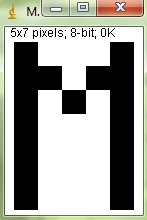```M = [...
1 0 0 0 1 ...
1 1 0 1 1 ...
1 0 1 0 1 ...
1 0 0 0 1 ...
1 0 0 0 1 ...
1 0 0 0 1 ...
1 0 0 0 1 ...
]';
```

The output of the network is calculated by inserting each of these vectors into `ann_FF_run`instead of the training vector `x` For example,

```y = ann_FF_run(T_odd,N,W);
disp(y)
```

Then I write the code that displays

• “Looks like T” if the network recognizes “T”
• “Looks like U” if the network recognizes “U”
• “I can’t recognise it” if the letter is not recognised by the network
```if (y(1)> 0.8 & y(2) < 0.2)
disp('Looks like T')
elseif (y(2)> 0.8 & y(1) < 0.2)
disp('Looks like U')
else
disp('I can''t recognise it')
end
```

Feeding `T_odd` gave me the output:

```    0.8798083
0.1333073

Looks like T
```

Feeding `U_odd` produces the output:

```    0.1351676
0.8113366

Looks like U
```

The letter `M` was not recognized:

```    0.2242181
0.8501858

I can't recognise it ```